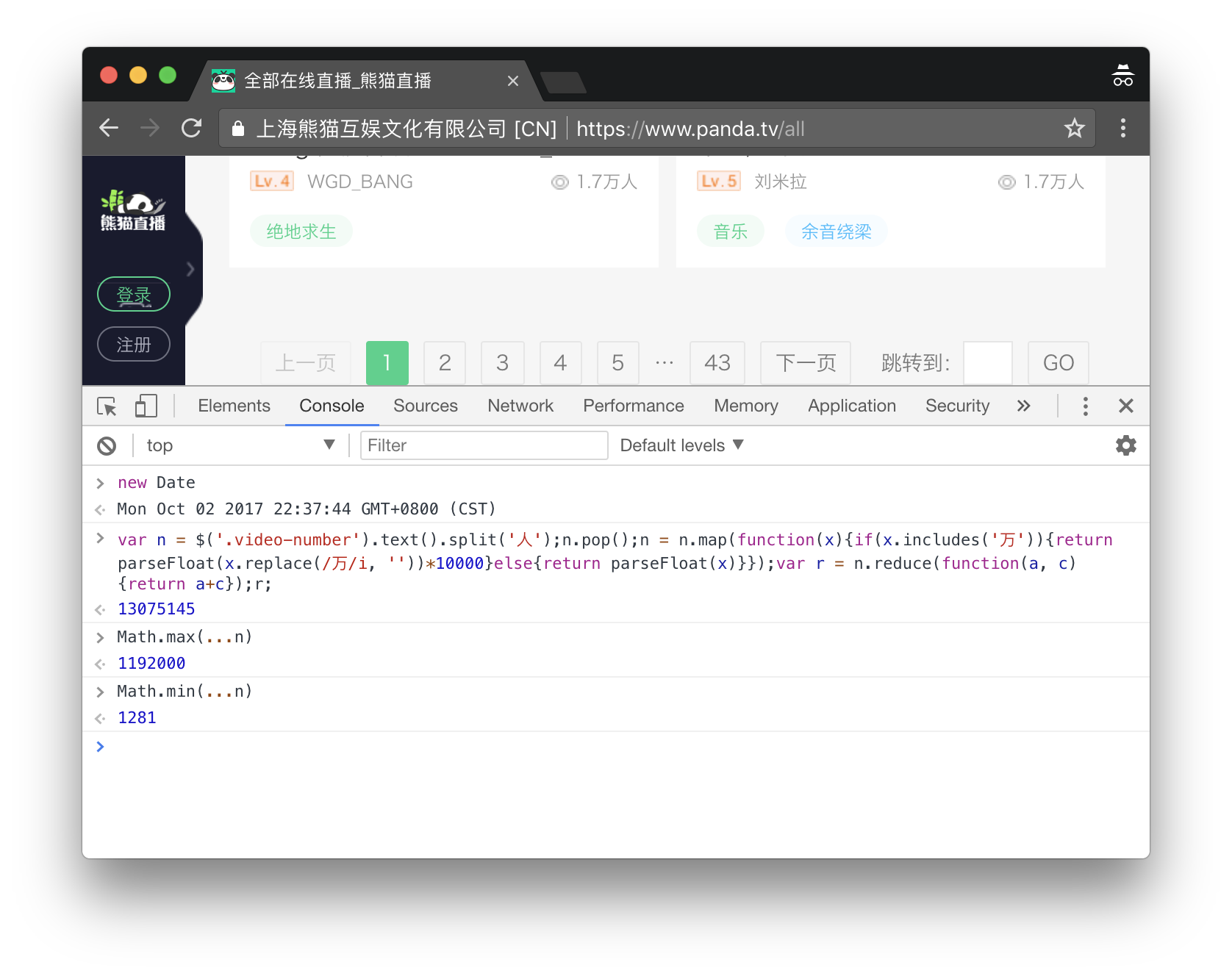Winner winner, chicken dinner!``````var n = \$('.video-number').text().split('人');
n.pop();
n = n.map(function(x) {
if (x.includes('万')) {
return parseFloat(x.replace(/万/i, '')) * 10000
} else {
return parseFloat(x)
}
});
var r = n.reduce(function(a, c) { return a + c });
r;
``````

``````var n = \$\$('.video-number')
.map(function(x) { return x.innerText })
.map(function(x) {
if (x.includes('万人')) {
return parseFloat(x.replace(/万人/i, '')) * 10000
} else {
return parseFloat(x.replace(/人/i, ''))
}
});
var r = n.reduce(function(a, c) { return a + c });
r;
``````

``````var n = \$\$('.dy-num').map(function(x){return x.innerText}).map(function(x){if(x.includes('万')){return parseFloat(x.replace(/万/, '')*10000)}else{return parseFloat(x)}});var r = n.reduce(function(a, c){return a + c});r;
``````

``````var n = \$\$('.js-num').map(function(x){return x.innerText}).map(function(x){if(x.includes('万')){return parseFloat(x.replace(/万/, '')*10000)}else{return parseFloat(x)}});var r = n.reduce(function(a, c){return a + c});r;
``````